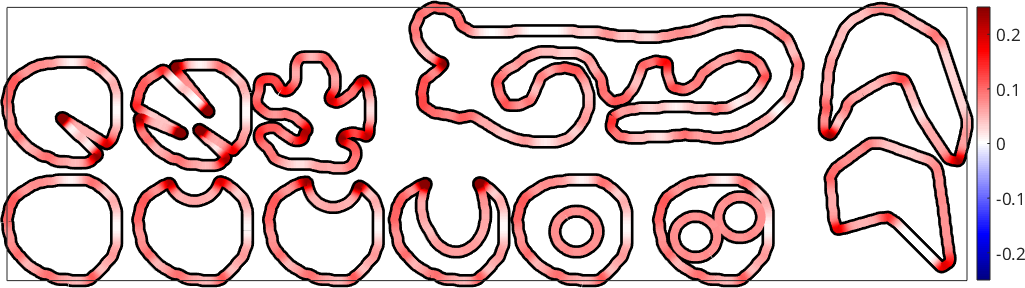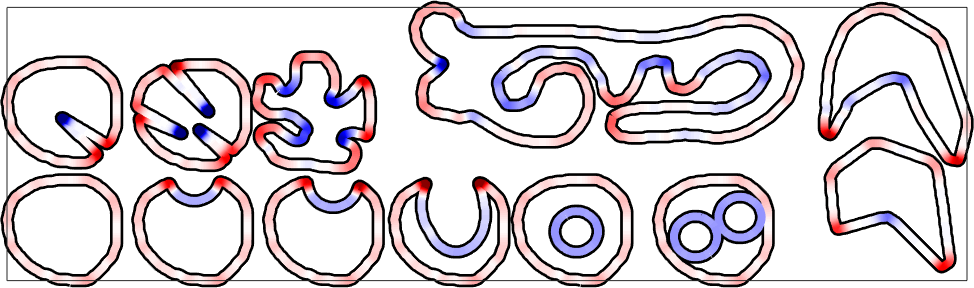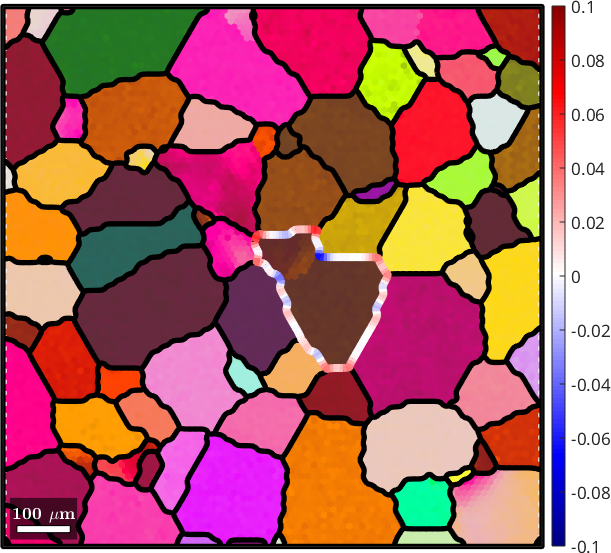Boundary Curvature edit page

The curvature of a curve is defined by fitting localy a circle and taking one over its radius. Hence, a straight line will have curvature 0 and a circle of radius $$2$$ will have constant curvature $$1/2$$ everywhere. Hence, the unit of the curvature computed in MTEX is one over the unit of the EBSD coordinates which is usually 1/µm. Let us demonstrate boundary curvature use some artifical grain shapes

Therefore, we first extract all boundary segments and colorize them according to their curvature.Note that all the curvature values are positive. This allways happens if the curvature is computed for multiple grains at one. If we consider single grains and compute the curvature of single grain boundaries the sign of the values indicates whether the grain boundary is convex for concav with respect to the specifc grain.Finally we illustrate the ussage of the curvature command at a real EBSD map.